# JAVA SE基础《七》 ---- JAVA案例

1、业务分析、随机生成一组中奖号码

2、让用户输入一组号码

3、判断中奖情况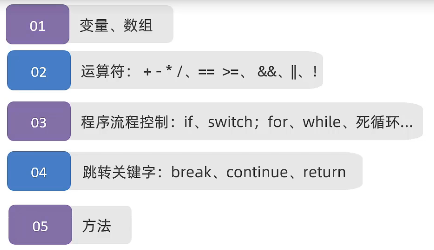目的2：积攒大家的代码量，以训练并提升大家的编程能力，编程思维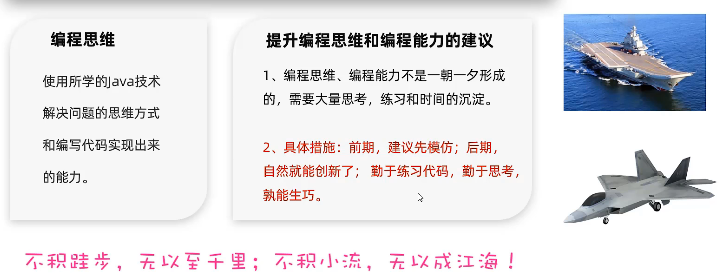• 方法是否需要接受数据？需要接受机票原价、当前月份、仓位类型；
• 方法是否需要返回数据？需要返回计算出的机票优惠价
• 方法内部：先使用if判断月份时旺季还是淡季，然后使用switch分支判断是头等舱还是经济舱
public class test {

public static void main(String[] args) {
double price = calculate(1000,8,"经济舱");
System.out.println("优惠价是："+ price);
}

public static double calculate(double price, int month, String type) {
// 1.判断当前月份是旺季还是淡季
if (month >= 5 && month <= 10) {
switch (type) {
case "头等舱":
price = price * 0.9;
break;
case "经济舱":
price *= 0.85;
break;
}
} else {
switch (type) {
case "头等舱":
price = price * 0.9;
break;
case "经济舱":
price *= 0.85;
break;
}
}
return price;
}
}

1、遇到需要通过判断数据在哪个区间，来决定执行哪个业务，应该用什么实现？

• 应该使用if分支结构实现。

2、遇到需要通过判断数据匹配哪个值，来决定执行哪个业务，应该用什么实现？

• 应该使用switch分支结构实现。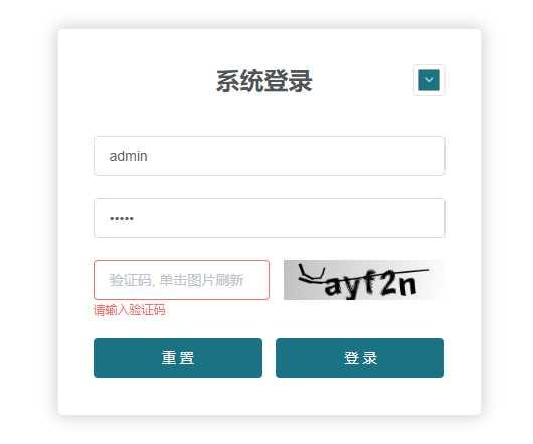案例 开发验证码

• 定义方法实现随机产生一个指定位数的验证码，每位可能是数字、大写、小写字母。

①方法是否需要接受数据？需要接收一个整数，控制生成验证码的位数。

②方法是否需要返回数据？需要返回生成的验证码。

③方法内部的业务：使用for循环依次生成每位随机字符，并使用一个String类的变量把每个字符连接起来，最后返回该变量即可。

package com.ctgu.demo;
import java.util.Random;

public class test {

public static void main(String[] args) {
System.out.println(createCode(5));
}
public static  String createCode(int n){
Random r = new Random();
String code = "";
for (int i = 0; i < n; i++) {
int type = r.nextInt(3);
switch (type){
case 0:
//随机一个数字字符
code += r.nextInt(10);
break;
case 1:
//随机一个大写字符 A 65 / 65+25
char ch1 = (char) (r.nextInt(26)+65);
code += ch1;
break;
case 2:
// 随机一个小写字符
char ch2 = (char) (r.nextInt(26)+97);
code += ch2;
break;
}

}
return  code;
}

}

1、随机验证码的核心实现逻辑是如何进行的？

• 定义一个String类型的变量存储验证码字符。
• 定义一个for循环，循环5次。
• 随机生成0|1|2的数据，依次代表当前位置要生成数字|大写字母|小写字母。
• 把0、1、2交给switch生成对应类型的随机字符，把字符交给String变量。
• 循环结束后，返回String类型的变量即是所求的验证码结果。

• 把6个评委的分数录入到程序中去 ----> 使用数组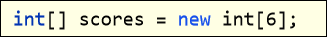• 遍历数组中每个数据，进行累加求和，并找出最高分、最低分。
• 按照分数的计算规则算出平均分。
package com.ctgu.demo;

import java.util.Random;
import java.util.Scanner;

public class test {

public static void main(String[] args) {
System.out.println(getAverageScore(6));
}
public static double getAverageScore(int number){
int[] scores = new int[number];
Scanner sc = new Scanner(System.in);
for (int i = 0; i < scores.length; i++) {
System.out.println("请您录入第" + (i+1) + "个评委的分数：");
int score = sc.nextInt();
scores[i] = score;
}
int sum = 0;
int max = scores;
int min = scores;

for (int i = 0; i < scores.length; i++) {
sum += scores[i];
if (max < scores[i])
max = scores[i];
if(min > scores[i])
min = scores[i];
}
return 1.0 * (sum - min - max) / (number - 2);
}

}

1、如何实现评委打分案例？

• 定义一个动态初始化的数组用于存储分数数据。
• 定义三个变量用于保存最大值、最小值和总和。
• 遍历数组中的每个元素，依次进行统计。
• 遍历结束后按照规则计算出结果即可。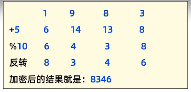分析

1、方法是否需要接受数据进行处理？需要接受四位数字密码，进行加密处理。

2、方法是否需要返回数据？需要返回加密后的结果。

3、方法内部的业务：将四位数密码差分成一个一个的数字，存入到数组中去，遍历数组中的每个数字，按照题目需求进行加密！最后，再把加密后的数字拼接起来返回即可！

package com.ctgu.demo;

import java.util.Random;
import java.util.Scanner;

public class test {

public static void main(String[] args) {
System.out.println("加密后的结果是："+encrpyt(1983));
}
public static String encrpyt(int number){
int[] numbers = split(number);
for (int i = 0; i < numbers.length; i++) {
numbers[i] = (numbers[i] + 5) % 10;
}
// 对数组反转，把对数据进行反转的操作交给一个独立的方法来完成
reverse(numbers);
String data = "";
for (int i = 0; i < numbers.length; i++) {
data += numbers[i];
}
return data;
}
private static void reverse(int[] numbers) {
for (int i = 0, j = numbers.length-1; i < j; i++,j--) {
// 交换i，j位置的值
int temp = numbers[j];
numbers[j] = numbers[i];
numbers[i] = temp;
}
}
private static int[] split(int number) {
int[] numbers = new int;
numbers = number/1000;
numbers = (number/100) % 10;
numbers = (number/10) % 10;
numbers = number % 10;
return numbers;
}
}

1、回顾数组元素的反转、交换是如何完成的？

• 反转数组，就是对数组中的元素，按照前后位置，依次交换数据。

2、如果一个方法里要做的事比较多，我们再开发中一般会怎么做？

• 一般会把多个事拆成多个方法去完成，也就是独立功能独立成一个方法。

1、方法是否需要接受数据进行处理？需要接受一个整型数组（原数组）

2、方法是否需要返回数据？需要返回一个新的、一摸一样的整型数组。

3、方法内部业务：创建一个长度一样的整型数组作为新数组，并把原数组的元素对应位置赋值给新数组，最终返回新数组即可。

package com.ctgu.demo;
import java.util.Random;
import java.util.Scanner;

public class test {

public static void main(String[] args) {
int[] arr = {11,22,33};
int[] arr2 = copy(arr);
printArray(arr2);
}
public static void printArray(int[] arr){
System.out.print("[");
for (int i = 0; i < arr.length; i++) {
System.out.print(i == arr.length - 1 ? arr[i] : arr[i] + ",");
}
System.out.println("]");
}
public static int[] copy(int[] arr){
int[] arr2 = new int[arr.length];
for (int i = 0; i < arr.length; i++) {
arr2[i] = arr[i];
}
return arr2;
}
}

1、数组的拷贝是什么意思？

• 创建出一个与原数组一模一样的数组。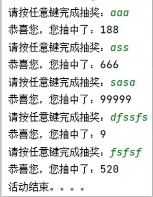分析

1、方法是否需要接受数据进行处理？需要接受一个数组，里面是5个金额，表示5个红包

2、方法是否需要返回数据？不需要

3、方法内部完成本需求的第一种方案：写一个for循环控制抽奖5次，每次抽奖，都从数组中随机找出一个金额，如果该金额不是0，则代表抽中，接着用0替换该位置处的金额，然后继续下一个粉丝的抽奖；如果抽中的金额发现是0，代表该位置处的红包之前被别人抽走了，则从新从数组中随机找出一个金额，继续判断！直至抽中的金额不是0！

package com.ctgu.demo;
import java.util.Random;
import java.util.Scanner;

public class test {
public static void main(String[] args) {
int[] moneys = {9,666,188,520,9999};
start(moneys);
}
public static void start(int[] moneys){

Scanner sc = new Scanner(System.in);
Random r = new Random();
// moneys = {9,666,188,520,99999}
for (int i = 1; i <= 5; i++) {
System.out.println("请您输入任意内容进行抽奖：");
sc.next(); // 等待用户输入内容，按了回车才往下走。

while (true) {
int index = r.nextInt(moneys.length); // 0-4
int money = moneys[index];

if(money != 0 ){
System.out.println("恭喜您，您抽中了红包，"+ money);;
moneys[index] = 0;
break;
}
}
}
System.out.println("活动结束。。");
}
}

4、方法内部完成本需求的第2种方案：先把数组里面的5个金额打乱顺序，打乱后的顺序就认为是中奖顺序；接着，写个for循环，执行5次，每次都提示抽奖；每次抽奖。都依次取出数组中的每个位置处的金额作为中奖金额即可。

package com.ctgu.demo;

import java.util.Random;
import java.util.Scanner;

public class test {

public static void main(String[] args) {
int[] moneys = {9, 666, 188, 520, 9999};
start(moneys);

}

public static void start(int[] moneys) {

Scanner sc = new Scanner(System.in);
Random r = new Random();
// moneys = {9,666,188,520,99999}
for (int i = 1; i < 5; i++) {
int index = r.nextInt(moneys.length); // 0-4
int temp = moneys[i];
moneys[i] = moneys[index];
moneys[index] = temp;
}
for (int i = 0; i < 5; i++) {
System.out.println("请您输入任意内容进行抽奖：");
sc.next(); // 等待用户输入内容，按了回车才往下走。

while (true) {
if (i < 5) {
System.out.println("恭喜您，您抽中了红包，" + moneys[i]);
break;
}
}

}
System.out.println("活动结束。。");

}
}

1、抢红包的实现方案有几种，哪种方式可能更好一些？

• 第1种：每次抽奖都从数组中，随机找出一个金额，如果该金额不是0，就输出该金额，然后用0替换该位置处的金额；如果该位置就是0，则重复上一步操作!
• 第2种：打乱奖金的顺序，再依次发给粉丝
• 　　遍历数组中的每个位置，每遍历一个位置，都随机一个索引值出来，让当前位置与该索引位置处的数据进行交换。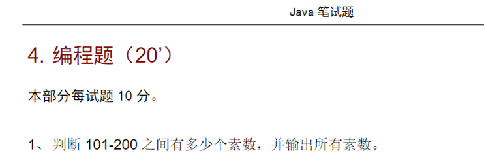1、方法是否需要接受数据进行处理？需要接受101以及200，以便找该区间中的素数。

2、方法是否需要返回数据？需要返回找到的素数个数。

3、方法内部的实现逻辑：使用for循环来产生如101到200之间的每个数；没拿到一个数，判断该数是否素数；判断规则是：从2开始遍历到该数的一半的数据，看是否有数据可以整除它，有则不是素数，没有则是素数；根据判定的结果来决定是否输出这个数据（是素数则输出）；最后还需要统计素数的个数并返回。

package com.ctgu.demo;
import java.util.Random;
import java.util.Scanner;
public class test {
public static void main(String[] args) {
// 目标：完成找素数
System.out.println("当前素数的个数是：" + search(101, 200));
}
public static int search(int start, int end){
int count = 0;
//start = 101 end = 200
// 1、定义一个for循环找到101到200之间的每个数据
for (int i = start; i < end; i++) {
// i = 101,102,....200

// 信号位思想
boolean flag = true;

// 2、判断当前1记住的这个数据是否是素数。
for (int j = 2; j <= i/2; j++) {
if(i % j == 0){
//当前记住的这个数据不是素数了
flag = false;
break;
}
}
// 3、根据判断的结果决定是否输出1当前记住的数据：是素数才输出展示。
if(flag){
System.out.println(i);
count++;
}
}
return count;
}
}

package com.ctgu.demo;

import java.util.Random;
import java.util.Scanner;

public class test {

public static void main(String[] args) {
// 目标：完成找素数
int count = 0;

OUT://为外部循环指定标签
for (int i = 101; i < 200; i++) {
// i = 101,102,....200
// 2、判断当前1记住的这个数据是否是素数。
for (int j = 2; j <= i / 2; j++) {
if (i % j == 0) {
//当前记住的这个数据不是素数了
continue OUT; //结束外部循环的当次执行
}
}
count ++;
System.out.println(i);
}
System.out.println("个数是：" + count);
}
}

package com.ctgu.demo;
import java.util.Random;
import java.util.Scanner;

public class test {

public static void main(String[] args) {
int count = 0;
for (int i = 101; i <= 200 ; i++) {
//i遍历到的当前数据是否是素数，是则输出，不是则不输出。
if(check(i)){
System.out.println(i);
count ++;
}
}
System.out.println("素数的个数是："+ count);
}
public static boolean check(int data){
for (int i = 2; i <= data/2 ; i++) {
if(data % i == 0){
return false; //不是素数
}
}
return true;
}
}

1、本次案例中是如何确定处该数是素数的，具体如何实现？

• 定义了flag标记位
• 遍历2到该数的一半的数据去判断是否有整除的数据，有则改变flag标记位的状态。
• 最终通过flag的状态判断是否是素数。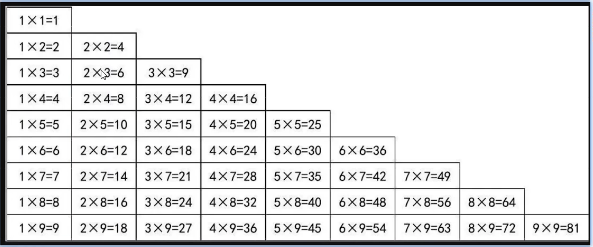package com.ctgu.demo;

public class test {

public static void main(String[] args) {
// 1、定义一个for循环控制打印多少行
for (int i = 1; i <= 9 ; i++) {
// 2、定义一个内部循环控制每行打印多少列。
for (int j = 1; j <= i; j++) {
// i 行 j列
System.out.print(j + "x" + i + "=" + (j * i) + "\t");
}
System.out.println(); //换行
}
}
}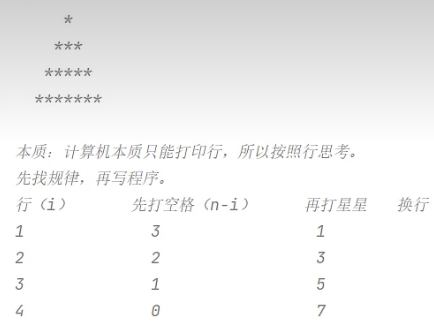package com.ctgu.demo;

public class test {

public static void main(String[] args) {
// 1、先定义一个循环控制打印多少行
int n = 4;
for (int i = 1; i <= 4 ; i++) {
// 2、控制打印多少个空格
for (int j = 1; j <= (n-i) ; j++) {
System.out.print(" ");
}
// 3、控制打印多少个星星
for (int j = 1; j <= (2*i - 1); j++) {
System.out.print("*");
}
// 4、换行
System.out.println();
}
}
}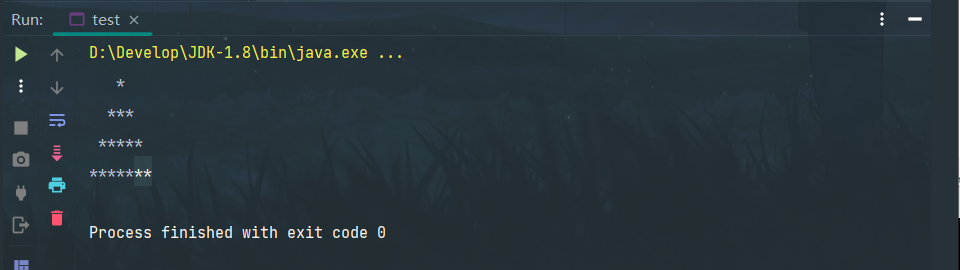二

package com.ctgu.demo;

public class test {

public static void main(String[] args) {
// 1、先定义一个循环控制打印多少行
int n = 4;
for (int i = 1; i <= 4 ; i++) {
// 2、控制打印多少个空格
for (int j = 1; j <= (n-i) ; j++) {
System.out.print(" ");
}
// 3、控制打印多少个星星
for (int j = 1; j <= (2*i - 1); j++) {
System.out.print(j % 2 == 0 ? " " : "*");
}
// 4、换行
System.out.println();
}
}
}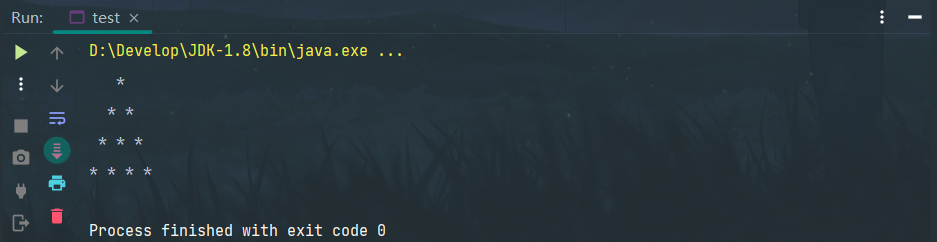1、业务分析、随机生成一组中奖号码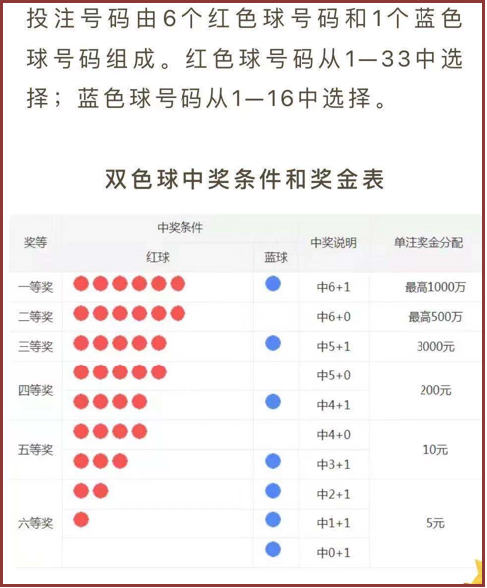总体实现步骤分析：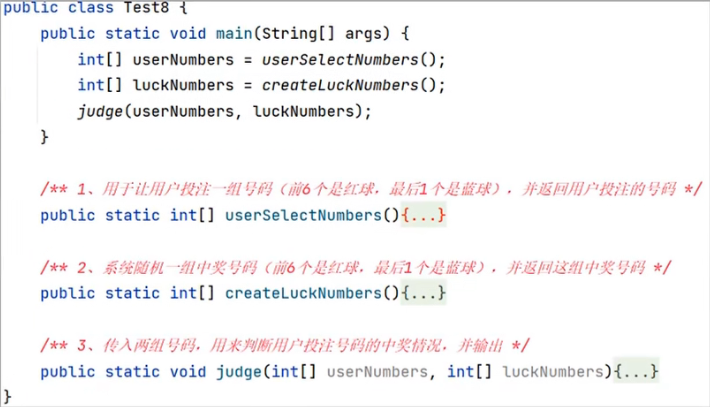注意6个红球号码的范围是1-33之间，且不能重复；1个篮球号码的范围在：1-16之间。

package com.ctgu.demo;
import java.util.Scanner;

public class test {

public static void main(String[] args) {
// 目标：完成双色球系统的开发。
int[] userNumbers = userSelectNumber();
printArray(userNumbers);
}
public static void printArray(int[] arr){
System.out.print("[");
for (int i = 0; i < arr.length; i++) {
System.out.print(i == arr.length - 1 ? arr[i] : arr[i] + ",");
}
System.out.println("]");
}

// 1、设计一个方法，用于让用户投注一组号码并返回（前6个是红球号码，最后1个是蓝球号码）
public static int[] userSelectNumber() {
// 2、创建一个整型数组，用于存储用户投注的7个号码
int[] numbers = new int;
// numbers = {0, 0, 0, 0, 0, 0, 0}
//            0  1  2  3  4  5  6
Scanner sc = new Scanner(System.in);
// 3、遍历前6个位置，让用户依次投注6个红球号码，存入
for (int i = 0; i < numbers.length - 1; i++) {
// i = 0 1 2 3 4 5
while (true) {
// 4、开始让用户为当前位置投注一个红球号码(1-33之间，不能重复)
System.out.println("请您输入第" + (i + 1) + "个红球号码（1-33之间，不能重复）:");
int number = sc.nextInt();

// 5、先判断用户数输入的红球号码是否在1-33之间
if (number < 1 || number > 33) {
System.out.println("对不起，您输入的红球号码不在1-33之间，请确认！");
} else {
// 号码是在1-33之间了。接着还需要继续判断这个号码是否重复，不重复才可以使用。
if (exist(numbers, number)) {
// number当前这个红球号码重复了
System.out.println("对不起，您当前输入的红球号码前面选择过，重复了，请确认！");
} else {
// number记住的这个号码没有重复了，就可以使用了。
numbers[i] = number;
break;
}
}
}
}
while (true) {
// 6.投注最后一个蓝球号码
System.out.println("请您输入最后1个蓝球号码（1-16）：");
int number = sc.nextInt();
if (number < 1 || number > 16) {
System.out.println("对不起，您输入的蓝球号码范围不对！");
} else {
numbers = number;
break;
}
}
return numbers;
}

private static boolean exist(int[] numbers, int number) {
// 需求：判断number这个数字是否在number数组中存在。
// numbers = {12,25,18,0,0,0,0}
// number = 26
for (int i = 0; i < numbers.length; i++) {
if(numbers[i] == 0){
break;
}
if(numbers[i] == number){
return true;
}
}
return false;
}
}

1、本次案例中是如何去保证用户投注的6个红球号码不重复的？

• 每次用户投注一个红球号码后，都去调用一个方法来判断这个号码是否已经选择过，如果选择过，让用户重新选号。

2、随机生成一组中奖号码

// 2、设计一个方法：随机一组中奖号码出来（6个红球号码，1个蓝球号码）
public static int[] createLuckNumbers(){
// 1、创建一个整型数组，用于存储7个号码
int[] numbers = new int;

Random r = new Random();
// 2、遍历6个位置处，依次随机一个红球号码存入（1-33不重复）
for (int i = 0; i < numbers.length; i++) {
// i = 0 1 2 3 4 5

while(true) {
// 3、为当前这个位置随机一个红球号码出来存入。1-33 ==》（0，32）+1
int number = r.nextInt(33) + 1;

// 4、判断这个号码是否之前出现过
if (!exist(numbers, number)) {
// number不重复
numbers[i] = number;
break; // 结束死循环，代表找到了当前这个位置的一个不重复的红球号码了。
}
}
}
// 3、录入一个蓝球号码，1-16
numbers = r.nextInt(16) + 1;
return numbers;
}

1、本次案例中是如何去保证随机的6个中奖的红球号码不重复的？

• 每次随机一个1-33之间的红球号码后，都去调用一个方法来判断这个号码是否已经出现过，如果出现过，让用户重新选号。

3、判断中奖情况

 public static void judge(int[] userNumbers,int[] luckNumbers){
// userNumbers = {12, 14, 16, 18, 23, 26, 8}
// luckNumbers = {16, 17, 18, 19, 26, 32, 8}

// 2、分别定义两个变量用于记住红球命中了几个以及蓝球命中看几个
int redCount = 0;
int blueCount = 0;

// 先判断红球命中的数量
// 遍历用户投注的号码的前6个红球
for (int i = 0; i < userNumbers.length - 1; i++) {
// userNumbers[i]
// 开始遍历中奖号码的前6个红球号码，看用户当前选择的这个号码是否命中
for (int j = 0; j < luckNumbers.length; j++) {
if (userNumbers[i] == luckNumbers[j]){
redCount++;
break;
}
}
}
// 3、判断蓝球是否命中
blueCount = userNumbers == luckNumbers ? 1 : 0;
System.out.println("您命中的红球数量是："+redCount);
System.out.println("您命中的蓝球数量是："+blueCount);

// 4、判断中奖详情，并输出结果
if(redCount == 6 && blueCount == 1){
System.out.println("恭喜你，中奖1000万，可以开始享受人生了~~~");
} else if (redCount == 6 && blueCount == 0) {
System.out.println("恭喜你，中奖500万，可以稍微开始享受人生了~~~");
} else if (redCount == 5 && blueCount == 1) {
System.out.println("恭喜你，中奖3000元，可以出去吃顿小龙虾了~");
} else if (redCount == 5 && blueCount == 0 || redCount==4 && blueCount == 1) {
System.out.println("恭喜你,中了小奖，200元~");
} else if (redCount < 3 && blueCount == 1 ) {
System.out.println("中了5元~");
}else {
System.out.println("感谢你对福利事业做出的巨大贡献！");
}
}

package com.ctgu.demo;

import java.util.Random;
import java.util.Scanner;

public class test {

public static void main(String[] args) {
// 目标：完成双色球系统的开发。
int[] userNumbers = userSelectNumber();
System.out.println("您投注的号码：");
printArray(userNumbers);

int[] luckNumbers = createLuckNumbers();
System.out.println("中奖的号码：");
printArray(luckNumbers);

judge(userNumbers,luckNumbers);
}
public static void printArray(int[] arr){
System.out.print("[");
for (int i = 0; i < arr.length; i++) {
System.out.print(i == arr.length - 1 ? arr[i] : arr[i] + ",");
}
System.out.println("]");
}

// 1、设计一个方法，用于让用户投注一组号码并返回（前6个是红球号码，最后1个是蓝球号码）
public static int[] userSelectNumber() {
// 2、创建一个整型数组，用于存储用户投注的7个号码
int[] numbers = new int;
// numbers = {0, 0, 0, 0, 0, 0, 0}
//            0  1  2  3  4  5  6
Scanner sc = new Scanner(System.in);
// 3、遍历前6个位置，让用户依次投注6个红球号码，存入
for (int i = 0; i < numbers.length - 1; i++) {
// i = 0 1 2 3 4 5
while (true) {
// 4、开始让用户为当前位置投注一个红球号码(1-33之间，不能重复)
System.out.println("请您输入第" + (i + 1) + "个红球号码（1-33之间，不能重复）:");
int number = sc.nextInt();

// 5、先判断用户数输入的红球号码是否在1-33之间
if (number < 1 || number > 33) {
System.out.println("对不起，您输入的红球号码不在1-33之间，请确认！");
} else {
// 号码是在1-33之间了。接着还需要继续判断这个号码是否重复，不重复才可以使用。
if (exist(numbers, number)) {
// number当前这个红球号码重复了
System.out.println("对不起，您当前输入的红球号码前面选择过，重复了，请确认！");
} else {
// number记住的这个号码没有重复了，就可以使用了。
numbers[i] = number;
break;
}
}
}
}
while (true) {
// 6.投注最后一个蓝球号码
System.out.println("请您输入最后1个蓝球号码（1-16）：");
int number = sc.nextInt();
if (number < 1 || number > 16) {
System.out.println("对不起，您输入的蓝球号码范围不对！");
} else {
numbers = number;
break;
}
}
return numbers;
}

private static boolean exist(int[] numbers, int number) {
// 需求：判断number这个数字是否在number数组中存在。
// numbers = {12,25,18,0,0,0,0}
// number = 26
for (int i = 0; i < numbers.length; i++) {
if(numbers[i] == 0){
break;
}
if(numbers[i] == number){
return true;
}
}
return false;
}

// 2、设计一个方法：随机一组中奖号码出来（6个红球号码，1个蓝球号码）
public static int[] createLuckNumbers(){
// 1、创建一个整型数组，用于存储7个号码
int[] numbers = new int;

Random r = new Random();
// 2、遍历6个位置处，依次随机一个红球号码存入（1-33不重复）
for (int i = 0; i < numbers.length; i++) {
// i = 0 1 2 3 4 5

while(true) {
// 3、为当前这个位置随机一个红球号码出来存入。1-33 ==》（0，32）+1
int number = r.nextInt(33) + 1;

// 4、判断这个号码是否之前出现过
if (!exist(numbers, number)) {
// number不重复
numbers[i] = number;
break; // 结束死循环，代表找到了当前这个位置的一个不重复的红球号码了。
}
}
}
// 3、录入一个蓝球号码，1-16
numbers = r.nextInt(16) + 1;
return numbers;
}

// 3、设计一个方法，用于判断用户的中奖情况
public static void judge(int[] userNumbers,int[] luckNumbers){
// userNumbers = {12, 14, 16, 18, 23, 26, 8}
// luckNumbers = {16, 17, 18, 19, 26, 32, 8}

// 2、分别定义两个变量用于记住红球命中了几个以及蓝球命中看几个
int redCount = 0;
int blueCount = 0;

// 先判断红球命中的数量
// 遍历用户投注的号码的前6个红球
for (int i = 0; i < userNumbers.length - 1; i++) {
// userNumbers[i]
// 开始遍历中奖号码的前6个红球号码，看用户当前选择的这个号码是否命中
for (int j = 0; j < luckNumbers.length; j++) {
if (userNumbers[i] == luckNumbers[j]){
redCount++;
break;
}
}
}
// 3、判断蓝球是否命中
blueCount = userNumbers == luckNumbers ? 1 : 0;
System.out.println("您命中的红球数量是："+redCount);
System.out.println("您命中的蓝球数量是："+blueCount);

// 4、判断中奖详情，并输出结果
if(redCount == 6 && blueCount == 1){
System.out.println("恭喜你，中奖1000万，可以开始享受人生了~~~");
} else if (redCount == 6 && blueCount == 0) {
System.out.println("恭喜你，中奖500万，可以稍微开始享受人生了~~~");
} else if (redCount == 5 && blueCount == 1) {
System.out.println("恭喜你，中奖3000元，可以出去吃顿小龙虾了~");
} else if (redCount == 5 && blueCount == 0 || redCount==4 && blueCount == 1) {
System.out.println("恭喜你,中了小奖，200元~");
} else if (redCount < 3 && blueCount == 1 ) {
System.out.println("中了5元~");
}else {
System.out.println("感谢你对福利事业做出的巨大贡献！");
}
}

}

1、本次案例中是如何去统计用户投注的红球的命中数量的？

• 遍历用户选择的每个红球号码，每遍历一个红球号码时，都去遍历中奖号码数组中的全部红球号码，看当前选的红球号码是否在中奖号码中存在，存在则红球命中数量加1
posted @ 2023-07-18 18:52  小王子C  阅读(71)  评论(0编辑  收藏  举报